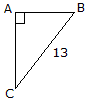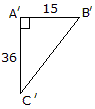# Engineering Mechanics - General Principles - Discussion

### Discussion :: General Principles - General Questions (Q.No.7)

7.Determine the length of side AB if right angle ABC is similar to right angle A'B'C':

 [A]. AB = 5.42 [B]. AB = 3 [C]. AB = 5 [D]. AB = 4

Explanation:

No answer description available for this question.

 Naveen said: (Dec 10, 2010) B'C'=(36^2+15^2)^1/2 B'C'=39 From similar Triangles AB/A'B' =13/39 AB=5

 Saurabh Khatri said: (Jun 7, 2011) Here. C'B'=39. But CB=13. So CB=1/3 part of C'B'. Then AB=1/3 part of A'B' (triangles are similar). So AB=5.

 M.Prakash said: (Jul 4, 2011) How to solve this problem explain?

 Kaushik Gamit said: (Oct 5, 2011) (36^2+15^2)^1/2=39 According to A'B'C' tanx=36/15 x=67.38 is equals to

 Ashwin said: (Nov 28, 2011) B'C'=(36^2+15^2)^1/2 B'C'=39 From similar Triangles BC/B'C' =13/39 =0.33333 AB=A'B' x 0.333 = 5.

 Hamid Alawi said: (Jun 21, 2013) B'C' = 36^2 + 15^2 = 1521 square root = 39. 39/ 13 =3. BC =13. Now we get AC . 36/3 =12. 13 + 12 = 25 square root of 25 = 5.

 Shruti said: (Dec 18, 2013) Is they are equilateral triangle?

 Nayan said: (Apr 21, 2015) (B'C')^2 = (A'C')^2+(A'B')^2. B'C' = sqrt[(36)^2+(15)^2]. B'C' = 39. From Similar Triangles, AB/A'B' = BC/B'C'. AB = BC*A'B'/B'C'. AB = 13*15/39. AB = 5.

 Aditya Kashyap said: (Apr 28, 2020) b'c'= square root 36^2+15^2=39 we get; B'C'\BC=AC\A'C' THEN WE GET AC=13*36 \39=12. c=12. AB= √ 13^2-12^2=5 ANS.